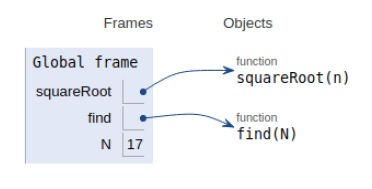# Python Program for Maximum height when coins are arranged in a triangle

Problem statement − We are given N coins where we need to arrange them in form of a triangle, i.e. in the first row will have 1 coin, the second row will have 2 coins and so on, we need to display the maximum height that can be achieved by the help N coins.

Now let’s observe the solution in the implementation below −

## Example

Live Demo

# squareroot
def squareRoot(n):
# initial approximation
x = n
y = 1
e = 0.000001 # allowed error
while (x - y > e):
x = (x + y) / 2
y = n/x
return x
# max height
def find(N):
# calculating portion of the square root
n = 1 + 8*N
maxH = (-1 + squareRoot(n)) / 2
return int(maxH)
# main
N = 17
print("Maximum height is :",find(N))

## Output

Maximum height is : 5All the variables are declared in the local scope and their references are seen in the figure above.

## Conclusion

In this article, we have learned about how we can make a Python Program for Maximum height when coins are arranged in a triangle.